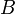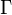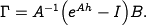# Errata: Missing factor of B in displayed equation after equation (5.28)

In the displayed equation after equation (5.28), theterm is missing from the expression for. The correct equation is